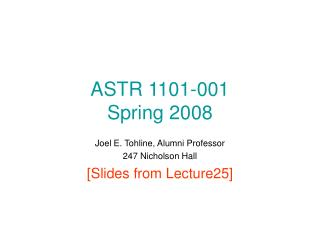DownloadDownload PresentationASTR 1101-001 Spring 2008

# ASTR 1101-001 Spring 2008

Télécharger la présentation## ASTR 1101-001 Spring 2008

- - - - - - - - - - - - - - - - - - - - - - - - - - - E N D - - - - - - - - - - - - - - - - - - - - - - - - - - -
##### Presentation Transcript

1. ASTR 1101-001Spring 2008 Joel E. Tohline, Alumni Professor 247 Nicholson Hall [Slides from Lecture25]

2. Chapter 8: Principal Topics • How old is the Solar System? • Nebular Hypothesis + Planetesimals + Core Accretion: A model that explains how the solar system acquired its key structural properties. • Directions and orientations of planetary orbits • Relative locations of terrestrial and Jovian planets • Size and compositions of planets • Observational evidence for extrasolar planets

3. How old is the Solar System? • Radioactive dating • First, let’s discuss the idea of radioactive isotopes of atomic elements

4. How old is the Solar System? • Radioactive dating • First, let’s discuss the idea of (radioactive & non-radioactive) isotopes of atomic elements

5. Chemical Elements & Their Isotopes Courtesy of:http://atom.kaeri.re.kr/

6. Chart of Nuclides

7. Chart of Nuclides

8. Chart of Nuclides

9. Chart of Nuclides

10. Chart of Nuclides

11. Chart of Nuclides

12. Chart of Nuclides

13. Chart of Nuclides 14 C 6 + 8 = 14

14. How old is the Solar System? • Radioactive dating • First, let’s discuss the idea of (radioactive & non-radioactive) isotopes of atomic elements • Now let’s discuss radioactivity and the concept of “half-life” • “The half-life of an isotope is the time interval in which one-half of the nuclei decay.” [See Box 8-1 in the textbook.] • http://www.colorado.edu/physics/2000/isotopes/radioactive_decay3.html

16. Color Indicates Approximate “Half-Life”

17. Some more precise “Half-Life” values

18. Some more precise “Half-Life” values

19. Some more precise “Half-Life” values

20. Decay of 14C to 14N 14C t1/2 = 5730 years 14N

21. Some more precise “Half-Life” values

22. Decay of 87Rb to 87Sr t1/2 = 47 billion years

23. Some more precise “Half-Life” values

24. Decay of 238U to 206Pb 238U t1/2 = 4.5 billion years 206Pb

25. Radioactive Decay • As the abundance of the radioactive isotope(for example, 14C) decreases steadily over time, the abundance of the final stable isotope(for example, 14N) steadily increases.

26. 14C Dating • Suppose an archeologist digs up a primitive weapon made partly of wood and determines that the wood contains an isotopic abundance ratio 14N/14C = 3. How old is the weapon if we assume that, originally, the wood contained no 14N ?

27. 14C Dating 14N 14C

28. 14C Dating At what time does the abundance ratio 14N/14C = 3 ? 14N 14C

29. 14C Dating At what time does the abundance ratio 14N/14C = 3 ? 14N 14C

30. 14C Dating At what time does the abundance ratio 14N/14C = 3 ? 14N 14C Age of wood = 2 t1/2 = 2 x (5730 yrs) = 11,460 yrs

31. 14C Dating • Suppose an archeologist digs up a primitive weapon made partly of wood and determines that the wood contains an isotopic abundance ratio 14N/14C = 3. How old is the weapon if we assume that, originally, the wood contained no 14N ? • ANSWER: 11,460 years • In practice, the ‘dating’ technique is messier than this, but this should give you a general idea of how the radioactive dating technique works.

32. 14C Dating • Suppose an archeologist digs up a primitive weapon made partly of wood and determines that the wood contains an isotopic abundance ratio 14N/14C = 3. How old is the weapon if we assume that, originally, the wood contained no 14N ? • ANSWER: 11,460 years • In practice, the ‘dating’ technique is messier than this, but this should give you a general idea of how the radioactive dating technique works.

33. 238U Dating • Suppose an astronomer discovers a meteorite and determines that the meteorite contains an isotopic abundance ratio 206Pb/238U = 1. How old is the meteorite if we assume that, when it originally formed, the meteorite contained no 206Pb ?

34. Atomic Bombs • Rely on spontaneous fission (radioactive decay) of heavy nuclei, such as Uranium and Plutonium • A ‘critical mass’ of fissionable material will explode because the decay products from spontaneous fission strike nearby nuclei and induce those nuclei to fission  runaway chain reaction • First atomic bombs were constructed during World War II in the so-called ‘Manhattan Project’ centered at Los Alamos, NM http://www.lanl.gov/history/